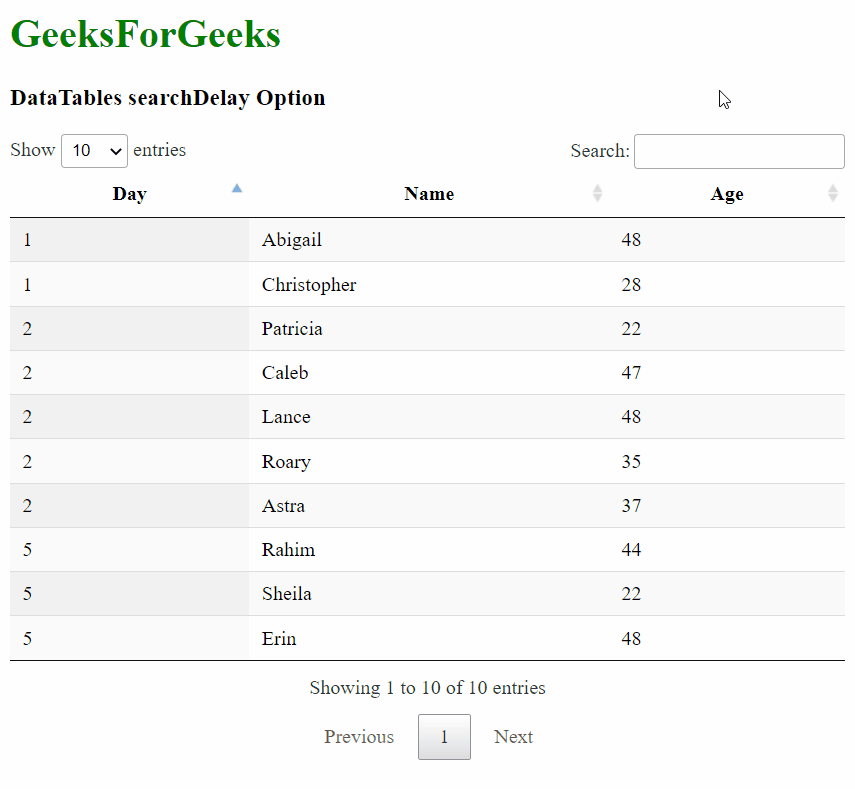Related Articles

# DataTables searchDelay Option

• Last Updated : 13 Jul, 2021

DataTables is a jQuery plugin that can be used for adding interactive and advanced controls to HTML tables for the webpage. This also allows the data in the table to be searched, sorted, and filtered according to the needs of the user. The DataTable also exposes a powerful API that can be further used to modify how the data is displayed.

The searchDelay option is used to specify the number of milliseconds after which the term entered the search box is searched in the table. This can be useful to reduce the number of redraws of the table and save processing power on slower systems. It accepts an integer value that denotes the number of milliseconds of the delay. The default value for client-side search is 0ms, which means that the value is searched and the results are displayed immediately. On the server-side, the delay is set to a default of 400ms to reduce the number of calls to the server.

Note: This affects only the global search box of the DataTable. The API methods like search() are not affected by changing this option.

Syntax:

`{ searchDelay: value }`

Parameters: This option has a single value as mentioned above and described below.

• value: This is an integer value that specifies the number of milliseconds after which the search is executed.

The example below illustrates the use of this option.

Example 1: In this example, we set the search delay to 2000 milliseconds.

## HTML

 ```<``html``>`` ` `<``head``>``  ````  ``<``script` `type``=``"text/javascript"` `          ``src``=``"https://code.jquery.com/jquery-3.5.1.js"``>``  ```` ` `  ````  ``<``link` `rel``=``"stylesheet"``        ``href``=``"https://cdn.datatables.net/1.10.23/css/jquery.dataTables.min.css"``>`` ` `  ````  ``<``script` `src``=``"https://cdn.datatables.net/1.10.23/js/jquery.dataTables.min.js"``>``  `````` ` `<``body``>``  ``<``h1` `style``=``"color: green;"``>``    ``GeeksForGeeks``  ````  ``<``h3``>DataTables searchDelay Option`` ` `  ````  ``<``table` `id``=``"tableID"` `class``=``"display nowrap"``>``    ``<``thead``>``      ``<``tr``>``        ``<``th``>Day``        ``<``th``>Name``        ``<``th``>Age``      ````    ````    ``<``tbody``>``      ``<``tr``>``        ``<``td``>2``        ``<``td``>Patricia``        ``<``td``>22``      ````      ``<``tr``>``        ``<``td``>2``        ``<``td``>Caleb``        ``<``td``>47``      ````      ``<``tr``>``        ``<``td``>1``        ``<``td``>Abigail``        ``<``td``>48``      ````      ``<``tr``>``        ``<``td``>5``        ``<``td``>Rahim``        ``<``td``>44``      ````      ``<``tr``>``        ``<``td``>5``        ``<``td``>Sheila``        ``<``td``>22``      ````      ``<``tr``>``        ``<``td``>2``        ``<``td``>Lance``        ``<``td``>48``      ````      ``<``tr``>``        ``<``td``>5``        ``<``td``>Erin``        ``<``td``>48``      ````      ``<``tr``>``        ``<``td``>1``        ``<``td``>Christopher``        ``<``td``>28``      ````      ``<``tr``>``        ``<``td``>2``        ``<``td``>Roary``        ``<``td``>35``      ````      ``<``tr``>``        ``<``td``>2``        ``<``td``>Astra``        ``<``td``>37``      ````    ````  ````  ``<``script``>`` ` `    ``// Initialize the DataTable``    ``\$(document).ready(function () {``      ``\$('#tableID').DataTable({`` ` `        ``// Enable delaying of the search``        ``// to the given number of milliseconds``        ``searchDelay: 2000``      ``});``    ``}); ``  `````` ` ``

Output:Example 2: In this example, we set the search delay to 0 milliseconds, which means that a search is instant.

## HTML

 ```<``html``>`` ` `<``head``>``  ````  ``<``script` `type``=``"text/javascript"` `          ``src``=``"https://code.jquery.com/jquery-3.5.1.js"``>``  ```` ` `  ````  ``<``link` `rel``=``"stylesheet"``        ``href``=``"https://cdn.datatables.net/1.10.23/css/jquery.dataTables.min.css"``>`` ` `  ````  ``<``script` `src``=``          ``"https://cdn.datatables.net/1.10.23/js/jquery.dataTables.min.js"``>``  `````` ` `<``body``>``  ``<``h1` `style``=``"color: green;"``>``    ``GeeksForGeeks``  ````  ``<``h3``>DataTables searchDelay Option`` ` `  ````  ``<``table` `id``=``"tableID"` `class``=``"display nowrap"``>``    ``<``thead``>``      ``<``tr``>``        ``<``th``>Day``        ``<``th``>Name``        ``<``th``>Age``      ````    ````    ``<``tbody``>``      ``<``tr``>``        ``<``td``>2``        ``<``td``>Patricia``        ``<``td``>22``      ````      ``<``tr``>``        ``<``td``>2``        ``<``td``>Caleb``        ``<``td``>47``      ````      ``<``tr``>``        ``<``td``>1``        ``<``td``>Abigail``        ``<``td``>48``      ````      ``<``tr``>``        ``<``td``>5``        ``<``td``>Rahim``        ``<``td``>44``      ````      ``<``tr``>``        ``<``td``>5``        ``<``td``>Sheila``        ``<``td``>22``      ````      ``<``tr``>``        ``<``td``>2``        ``<``td``>Lance``        ``<``td``>48``      ````      ``<``tr``>``        ``<``td``>5``        ``<``td``>Erin``        ``<``td``>48``      ````      ``<``tr``>``        ``<``td``>1``        ``<``td``>Christopher``        ``<``td``>28``      ````      ``<``tr``>``        ``<``td``>2``        ``<``td``>Roary``        ``<``td``>35``      ````      ``<``tr``>``        ``<``td``>2``        ``<``td``>Astra``        ``<``td``>37``      ````    ````  ```` ` `  ``<``script``>`` ` `    ``// Initialize the DataTable``    ``\$(document).ready(function () {``      ``\$('#tableID').DataTable({`` ` `        ``// Prevent any delay of the search``        ``// by setting the value to 0``        ``searchDelay: 0``      ``});``    ``}); ``  `````` ` ``

Output: